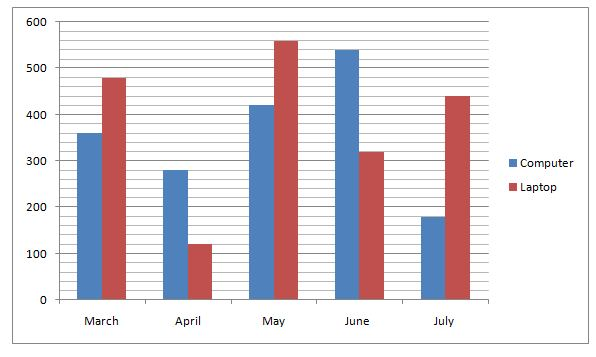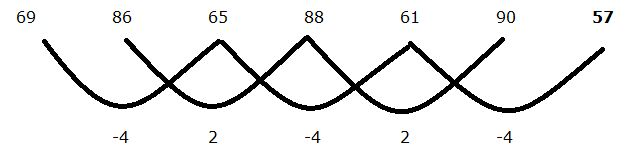# IBPS RRB PO Prelims Quantitative Aptitude Questions 2019 (Day-11)

Dear Aspirants, Our IBPS Guide team is providing new series of Quantitative Aptitude Questions for IBPS RRB PO Prelims 2019 so the aspirants can practice it on a daily basis. These questions are framed by our skilled experts after understanding your needs thoroughly. Aspirants can practice these new series questions daily to familiarize with the exact exam pattern and make your preparation effective.

Check here for IBPS RRB Prelims Mock Test 2019

[WpProQuiz 6719]

Directions (01 – 05): Find out the wrong number in the following number series.

1) 19, 235, 260, 324, 335, 341

a) 341

b) 235

c) 260

d) 335

e) 324

2) 69, 86, 65, 88, 61, 90, 56

a) 86

b) 61

c) 56

d) 88

e) 90

3) 13, 94, 45, 70, 60, 62

a) 94

b) 60

c) 70

d) 62

e) 45

4) 23, 46, 92, 161, 252, 268

a) 46

b) 161

c) 252

d) 92

e) 268

5) 27, 54, 162, 448, 3240, 19440

a) 54

b) 3240

c) 162

d) 448

e) 19440

Directions (6 – 10): Study the following information carefully and answer the questions given below.

The given bar graph shows the number of computers and laptops sold in five different months.6) What is the average number of laptops sold in all the months together?

a) 364

b) 384

c) 374

d) 368

e) 352

7) What is the ratio of the number of computer sold in July and May together to the number of laptop sold in April and June together?

a) 13: 10

b) 15: 11

c) 17: 13

d) 18: 13

e) None of these

8) What is the difference between the number of computer sold in March and June?

a) 120

b) 150

c) 180

d) 200

e) None of these

9) The number of computer sold in April is approximately what percent of the average number of computer sold in all the months together?

a) 71%

b) 75%

c) 79%

d) 82%

e) None of these

10) What is the sum of the computer and laptops sold in May and July together?

a) 1100

b) 1200

c) 1400

d) 1600

e) 1300

19 + 63 = 235

235 + 52 = 260

260 + 43 = 324

324 + 32 = 333

333 + 23 = 341

The wrong number is 335.

69             86             65             88             61             90             57

17             -21            23             -27            29             -33

4              2              4              2              4

Or,The wrong term is 56.

13 + 92 = 94

94 – 72 = 45

45 + 52 = 70

70 – 32 = 61

61 + 12 = 62

The wrong term is 60

23 + 23 * 1 = 46

46 + 23 * 2 = 92

92 + 23 * 3 = 161

161 + 23 * 4 = 253

253 + (23 * 5) = 368

The wrong term is 252.

27 * 1 = 27

27 * 2 = 54

54 * 3 = 162

162 * 4 = 648

648 * 5 = 3240

3240 * 6 = 19440

The wrong term is 448

Average = (480 + 120 +560 + 320 + 440) /5

= 384

Required ratio = (180 + 420):(120 + 320)

= 600: 440

= 15: 11

Difference = 540 – 360 = 180

Average number of computer = (360 + 280 + 420 + 540 + 180)/5

= 356

Required percentage = (280/356) * 100 = 79%